# MCAT Physical : Thin-Layer Chromatography

## Example Questions

### Example Question #1 : Thin Layer Chromatography

A new student is planning to use thin layer chromatography (TLC) for his research project. After setting up the apparatus the student forgets to place a lid on the TLC jar. He obtains poor results after running the TLC experiment. Which of the following can best explain his bad results?

The open system prevents the evaporation of the solvent on the TLC plate

The open system prevents the evaporation of the solvent in the solution

The open system evaporates the solvent in the solution

The open system evaporates the solvent on the TLC plate

The open system evaporates the solvent on the TLC plate

Explanation:

TLC is a laboratory technique commonly used to separate components of a mixture. Mixtures are placed on the TLC plate (stationary phase), which is then transferred to a jar containing the solvent. The solvent travels through the plate and carries components of the mixture along with it. Based on its properties, each component is dragged to different distances on the plate. The relative distances travelled by each component can be used for separation and identification.

It is important to place a lid on the jar because the solvent will be a volatile substance. An open system will allow for the solvent to evaporate from the TLC plate and reduce the amount of solvent travelling through the plate. The solvent in the solution will evaporate, but it is negligible and inconsequential to the data collected on the TLC plate.

### Example Question #2 : Thin Layer Chromatography

Which of the following can be determined from thin layer chromatography results?

I. Number of components in the mixture

II. The identity of the components

III. Polarity of components

II only

II and III

I, II, and III

I and III

I and III

Explanation:

Thin layer chromatography (TLC) is used to separate components in a mixture. Components are separated on a TLC plate because each component travels a different distance. The distance travelled depends on several factors. One of those factors is polarity; therefore, TLC can used to determine polarity of substances.

TLC is not useful for identifying substances. Other techniques such as NMR and IR spectroscopy are useful for identifying individual components. The Rf value of a compound can help identify it in a broad sense, but this technique provides only very rudimentary identification capabilities.

### Example Question #3 : Thin Layer Chromatography

After performing a TLC experiment, a researcher determines the Rf value of a component to be 2. He also notes that the solvent travelled a distance of 4cm on the plate. What can you conclude about this experiment?

There are multiple components in the mixture

The reported data does not seem valid

The solvent is not volatile

The component travelled a distance of 8cm

The reported data does not seem valid

Explanation:

To solve this problem we need to recall the definition of Rf.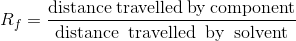The solvent is typically the most mobile substance on a TLC plate; therefore, it travels the farthest distance. This means that Rf is always less than one because the numerator of Rf is always smaller than the denominator. The question states that the Rf value is 2. Since this is greater than 1, the results don’t seem valid. This value indicates that the solute compounds were able to teravel farther on the TLC plate than the solvent (mobile phase).

### Example Question #4 : Thin Layer Chromatography

Compounds A and B are found in a mixture. Rf values for both compounds were calculated. Which of the following sets of Rf values would reflect the largest separation between A and B?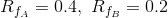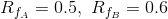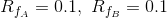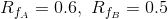Explanation:

Compounds A and B are in the same mixture; therefore, they will be separated using the same TLC plate. Recall the definition of Rf.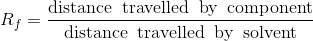Since the same TLC plate was used, the denominator for both compound A and B will be the same (the total distance travelled by solvent alone is the same for a TLC plate). To separate them effectively, compounds must have travelled different distances along the plate. The best separation occurs when the difference between the distances travelled by the compounds is greatest.

Let’s assume the denominator to be 1 for both compounds. This means that the numerator is equal to the Rf value. If we compare the given Rf values with each other we realize that Rf values of 0.4 and 0.2 will give the biggest difference between the distances travelled by compounds A and B (difference is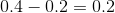); therefore, this will have the best separation.